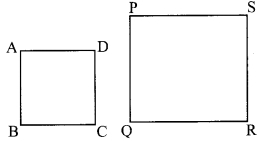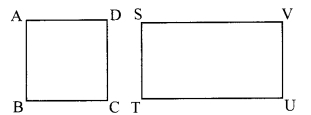# Maharashtra Board 9th Class Maths Part 2 Practice Set 3.5 Solutions Chapter 3 Triangles

Balbharti Maharashtra State Board Class 9 Maths Solutions covers the Practice Set 3.5 Geometry 9th Class Maths Part 2 Answers Solutions Chapter 3 Triangles.

## Practice Set 3.5 Geometry 9th Std Maths Part 2 Answers Chapter 3 Triangles

Question 1.
If ∆XYZ ~ ∆LMN, write the corresponding angles of the two triangles and also write the ratios of corresponding sides.
Solution:
∆XYZ ~ ∆LMN [Given]
∴ ∠X ≅ ∠L
∠Y ≅ ∠M >
∠Z ≅ ∠N [Corresponding angles of similar triangles]
$$\frac{\mathrm{XY}}{\mathrm{LM}}=\frac{\mathrm{YZ}}{\mathrm{MN}}=\frac{\mathrm{XZ}}{\mathrm{LN}}$$ [Corresponding sides of similar triangles]

Question 2.
In ∆XYZ, XY = 4 cm, YZ = 6 cm, XZ = 5 cm. If ∆XYZ ~ ∆PQR and PQ = 8 cm, then find the lengths of remaining sides of ∆PQR.
Solution:
∆XYZ ~ ∆PQR [Given]
∴ $$\frac{\mathrm{XY}}{\mathrm{PQ}}=\frac{\mathrm{YZ}}{\mathrm{QR}}=\frac{\mathrm{XZ}}{\mathrm{PR}}$$ [Corresponding sides of similar triangles]∴ PR = 10 cm
∴ QR = 12 cm, PR = 10cm

Question 3.
Draw a sketch of a pair of similar triangles. Label them. Show their corresponding angles by the same signs. Show the lengths of corresponding sides by numbers in proportion.
Solution:∆GHI ~ ∆STU

Maharashtra Board Class 9 Maths Chapter 3 Triangles Practice Set 3.5 Intext Questions and Activities

Question 1.
We have learnt that if two triangles are equiangular then their sides are in proportion. What do you think if two quadrilaterals are equiangular? Are their sides in proportion? Draw different figures and verify. Verify the same for other polygons. (Textbook pg no 50)
If two quadrilaterals are equiangular then their sides will not necessarily be in proportion.
Case 1: The two quadrilaterals are of the same type.Consider squares ABCD and PQRS.
∠A = ∠P, ∠B = ∠Q, ∠C = ∠R, ∠D = ∠S
$$\frac{\mathrm{AB}}{\mathrm{PQ}}=\frac{\mathrm{BC}}{\mathrm{QR}}=\frac{\mathrm{CD}}{\mathrm{RS}}=\frac{\mathrm{AD}}{\mathrm{PS}}$$

Case 2: The two quadrilaterals are of different types.Consider square ABCD and rectangle STUV.
∠A = ∠S, ∠B = ∠T, ∠C = ∠U, ∠D = ∠VScroll to Top